Open In App

# How to Convert Wide Dataframe to Tidy Dataframe with Pandas stack()?

We might sometimes need a tidy/long-form of data for data analysis. So, in python’s library Pandas there are a few ways to reshape a dataframe which is in wide form into a dataframe in long/tidy form. Here, we will discuss converting data from a wide form into a long-form using the pandas function stack(). stack() mainly stacks the specified index from column to index form. And it returns a reshaped DataFrame or even a series having a multi-level index with one or more new inner-most levels compared to the current DataFrame, these levels are created by pivoting the columns of the current dataframe and outputs a:

• Series: if the columns have a single level
• DataFrame: if the columns have multiple levels, then the new index level(s) is (are) taken from the specified level(s).

Syntax: DataFrame.stack(level=- 1, dropna=True)

Parameters –

• level : It levels to stack from the column axis to the index axis. It either takes an int, string or list as input value. And by default is set to -1.
• dropna : It asks whether to drop the rows into the resulting dataFrame or series in case they don’t have any values. It is of bool type and by default set to True.

Returns a stacked DataFrame or series.

Now, let’s start coding!

Case 1#:

Firstly, let’s start with a simple single-level column and a wide form of data.

## Python3

 `import` `pandas as pd`` ` `# Single level columns``df_single_level_cols ``=` `pd.DataFrame([[``74``, ``80``], [``72``, ``85``]],``                                    ``index``=``[``'Deepa'``, ``'Balram'``],``                                    ``columns``=``[``'Maths'``, ``'Computer'``])``print``(df_single_level_cols)`

Output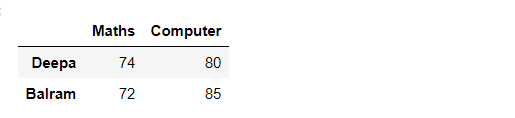Now, after we apply the stack() function Then we will get a dataframe with a single level column axis returns a Series:

## Python3

 `# Single level with stack()``df_single_level_cols.stack()`

Output: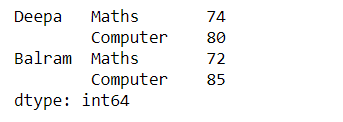Case 2#:

Let’s now try out with multi-level columns.

## Python3

 `# Simple Multi-level columns``multicol1 ``=` `pd.MultiIndex.from_tuples([(``'Science'``, ``'Physics'``),``                                       ``(``'Science'``, ``'Chemistry'``)])`` ` `df_multi_level_cols1 ``=` `pd.DataFrame([[``80``, ``64``], [``76``, ``70``]],``                                    ``index``=``[``'Deepa'``, ``'Balram'``],``                                    ``columns``=``multicol1)`` ` `print``(df_multi_level_cols1)`

Output: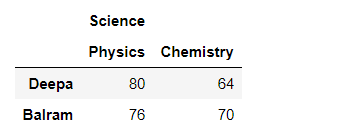After stacking the dataframe with a multi-level column axis:

## Python3

 `# Multi-level stacking with stack``df_multi_level_cols1.stack()`

Output: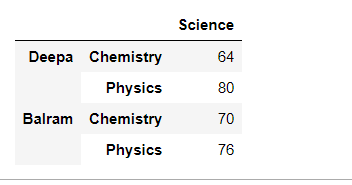Case 3#:

Now, let’s try with some missing values In the regular wide form, we will get the values as it is, since it has lesser value than the stacked forms:

## Python3

 `# Multi-level with missing values``multicol2 ``=` `pd.MultiIndex.from_tuples([(``'English'``, ``'Literature'``),``                                       ``(``'Hindi'``, ``'Language'``)])`` ` `df_multi_level_cols2 ``=` `pd.DataFrame([[``80``, ``75``], [``80``, ``85``]],``                                    ``index``=``[``'Deepa'``, ``'Balram'``],``                                    ``columns``=``multicol2)``df_multi_level_cols2`

Output: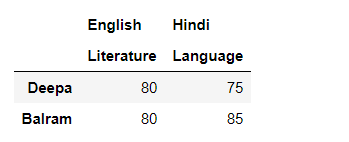But when we stack it,

We can have missing values when stacking a dataframe with multi-level columns, as the stacked dataframe typically has more values than the original dataframe. Missing values are filled with NaNs, like here in this example, the value for the English Language is not known so is filled with NaN.

## Python3

 `# Multi-level missing values as NaN``df_multi_level_cols2.stack()`

Output: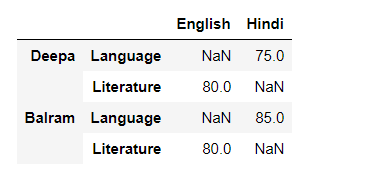Case 4#:

Apart from that, we can also contain the stacked values as per our preferences, hence by prescribing the stack the value to be kept. The first parameter actually controls which level or levels are stacked. Like,

## Python3

 `# Prescribing the level(s) to be stacked``df_multi_level_cols2.stack(``0``)`` ` `# The first parameter controls which level ``# or levels are stacked``df_multi_level_cols2.stack([``0``, ``1``])`

Output: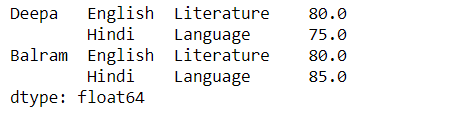Case 5#:

Now, finally let’s check what is the purpose of the dropna in stack(). For this, we will drop the rows which have completely NaN values. Let’s check the code for the regular result when the NaN values are included.

## Python3

 `# Dropping missing values``df_multi_level_cols3 ``=` `pd.DataFrame([[``None``, ``80``], [``77``, ``82``]],``                                    ``index``=``[``'Deepa'``, ``'Balram'``],``                                    ``columns``=``multicol2)``print``(df_multi_level_cols3)`` ` `# contains the row with all NaN values since,``# dropna=False``df_multi_level_cols3.stack(dropna``=``False``)``print``(df_multi_level_cols3)`

Output: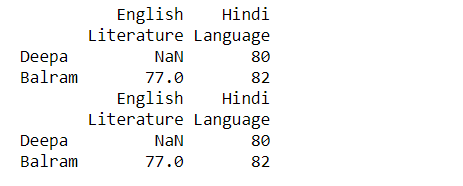Here, we can see in the Deepa index, the value for Literature is NaN when we are operating with dropna = False (it is including the NaN value as well)

Let’s check when we make the dropna = True( omits the complete NaN values row)

## Python3

 `# Drops the row with completely NaN values``df_multi_level_cols3.stack(dropna``=``True``)``print``(df_multi_level_cols3)`

Output: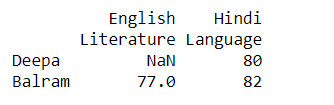So, here making dropna = False omits the Literature row as a whole since it was NaN completely.